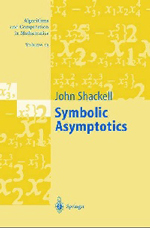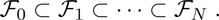Symbolic Asymptotics

J. R. Shackell
Springer Verlag, Berlin, Heidelberg, New York, 243 Seiten, 59,95 €

ISBN 3-540-21097-0

This book is the first text book about symbolic asymptotics, written by one of its major contributors. It deals with the question to calculate limits and asymptotic expansions of real functions symbolically.

The book contains the following chapters:
Chapter 1: Introduction. In the introduction the author explains the concepts of towers of fields, explog-functions etc., and shows by examples which algorithmic problems occur.
Chapter 2: Zero Equivalence. In this chapter zero equivalence of constants and of functions is considered. Schanuel’s Conjecture and Richardson’s Uniformity Conjecture are touched. Algorithms and examples are given. Modular methods and Hensel lifting are discussed. Systems of partial differential equations and differential ideals are introduced.
Chapter 3: Hardy Fields. The concept of Hardy fields is introduced, their closure properties and their relation to algebraic differential equations is discussed. Further properties of Hardy fields are investigated.
Chapter 4: Output Data Structures. Here asymptotic power series as well as multiseries which enable the use of different scales are introduced. Exponentials, logarithms, powers etc. of multiseries are treated. Finally the algebra of star products is investigated. Star products are generalizations of nested expansions and multiseries.
Chapter 5: Algorithms for Function Towers. To compute limits and asymptotic expressions, one regards functions as elements in a tower of Hardy fieldsProblems that occur in this treatment are discussed, and an algorithm for the exp-log case is given. The exp-log case is generalized by adding exponentials, integrals, algebraic entensions etc. Finally Cartesian representations are studied.
Chapter 6: Algebraic Differential Equations. Nested forms of Hardy field solutions of algebraic differential equations are discussed, and an algorithm to detect such solutions is given. Theorems about the number of cases and ideas to reduce the complexity are given. Finally sparse differential equations are considered.
Chapter 7: Inverse Functions. Until recently it was unknown whether the inverse of an exp-log function is necessarily asymptotic to an exp-log function. That this is not the case was discovered by Shackell in 1993. However, inverses of Hardy field members that tend to infinity belong to a Hardy field, again. In this chapter the inversion of nested expansions is covered, and multiseries of inverse functions are discussed.
Chapter 8: Implicit Functions. In this chapter asymptotic series of implicit functions are considered.
Chapter 9: Star-Product Expansions. The rewriting of exp-log expressions into standard star expansion form is discussed. Growth classes in Hardy fields are introduced.
Chapter 10: Oscillating Functions. Oscillating functions are never members of Hardy fields. Hence this chapter deals with different methods for this purpose. The book finishes with an example.

Many algorithms and examples are discussed in the book. I would have been interested to read in detail which of these algorithms are implemented in an existing computer algebra system like Maple. It is not clearly stated which parts of the book are covered by Maple’s asympt command. However, on pp. 91–92 the author informs about Dominik Gruntz’s limit implementation in Maple, and announces a current update process of asympt by Bruno Salvy.

The book contains a wealth of information on symbolic asymptotics. It fills a gap in the literature and should be found in every library. The user of a computer algebra system who is interested to understand the output of a command like Maple’s asympt finds much interesting information. Actually many users might not even be aware about the complexity of such a command.

Rezension: Wolfram Koepf (Kassel) aus Computeralgebra-Rundbrief, Nr. 37 - Oktober 2005